HPS 0410 Einstein for Everyone

Back to main course page

# Atoms and the Quantum

John D. Norton
Department of History and Philosophy of Science
University of Pittsburgh

This document is a duplication of "Atoms Entropy Quanta: Einstein's Statistical Physics of 1905," found in the Goodies section of mywebsite at www.pitt.edu/~jdnorton/goodies .

Einstein's work in statistical physics of 1905 is unified by a single insight: Physical systems that consist of many, spatially localized, independent micro-components have distinctive macro-properties. These macro-properties provide a signature that reveals the system's microscopic nature. Einstein used this insight in two ways. It enabled him to treat many, apparently distinct systems alike, simply because their micro-components are localized and independent. And he used the measurable macro-signature to reveal the micro-constitution of physical systems. In the case of heat radiation, the result was revolutionary.

## 1. The Three Statistical Papers of 1905

In his annus mirabilis of 1905, Einstein published three papers in statistical physics that appeared to be only loosely connected. They were:

Einstein's doctoral dissertation
"A New Determination of Molecular Dimensions"
Buchdruckerei K. J. Wyss, Bern, 1905. (30 April 1905)
Also: Annalen der Physik, 19(1906), pp. 289-305.
Einstein used known physical properties of sugar solution (viscosity, diffusion) to determine the size of sugar molecules.

"Brownian motion paper."
"On the motion of small particles suspended in liquids at rest required by the molecular-kinetic theory of heat."
Annalen der Physik, 17(1905), pp. 549-560.(May 1905; received 11 May 1905)
Einstein predicted that the thermal energy of small particles would manifest as a jiggling motion, visible under the microscope.

"Light quantum/photoelectric effect paper"
"On a heuristic viewpoint concerning the production and transformation of light."
Annalen der Physik, 17(1905), pp. 132-148.(17 March 1905)
Einstein inferred from the thermal properties of high frequency heat radiation that it behaves thermodynamically as if constituted of spatially localized, independent quanta of energy.

These three papers were intimately connected by a single insight that Einstein used and developed as the content of the papers unfolded. Take a system that consists of very many, spatially localized, independent microscopic components. That constitution can be read from the thermal properties of the system, as long as one knows how to read the signs. The most familiar example is a very dilute kinetic gas; its component molecules move independently. This constitution is directly expressed in the fact that the pressure, temperature and volume of the gas conforms to the ideal gas law.

Einstein was not the first to see these sort of possibilities. However he used them with greater fluidity and reach than ever before.

## 2. A Mini-Tutorial on Ideal Gases

For a very gentle warm up exercise, see "How big is an atom?"

To illustrate this insight, let us look at this most familiar case of ideal gases. This is the case of most ordinary gases, just like the air, when they are at ordinary temperatures that are not too cold and pressures that are not too high, so that they remain very dilute.Here is an ideal gas trapped in a cylinder by a weighted piston. That it obeys the ideal gas law means that the following calculation always works. Take the pressure P and multiply it by the volume V of the gas. Whatever you get will always be exactly the same as what you get when you take the number of molecules n, multiply it by Boltzmann's constant k and the temperature T. Or, to put it more simply: PV = nkT

This result is so simple that it is easy to miss what is quite remarkable about it. What is remarkable is exactly that it is so simple. Gases come in many different forms. We might have a very light gas like helium, the gas used to lift balloons, whose molecules are little spheres. Or we might have a denser gas like the oxygen of the air, whose molecules are dumbbell shaped. Or we might have a vaporized liquid, like water vapor, whose molecules are shaped something like little Mickey Mouse heads. In every case, the same law holds, even if the oxygen or water vapor are mixed up with another gas like nitrogen in the air. Yet nothing in the law takes note of all these differences. All that enters the law are the the volume, the temperature, the number of molecules and a single universal constant, Boltzmann's constant k. From them, using a little easy arithmetic, the law tells you what the gas pressure P will be.

How can the ideal gas law do this? It can do it because the truth of the law does not depend upon the detailed physical properties of the gas. Rather it depends only on a single fact shared by all dilute gases: they consist of may independent spatially localized molecules. The law needs this and nothing more; as a result it does not need to ask if the gas molecules are heavy or light, this shape or that; or even if the molecules are alone in space or surrounded by molecules of another type. This fact also foreshadows the far broader application of the ideal gas law than just to ideal gases.

Exactly how this law comes about is a somewhat technical issue, although not that technical. In its very simplest form it goes like this. The single most important result of the statistical physics of Maxwell and Boltzmann for a thermal systems is that the probability that one of its molecules is in some state is fixed by that state's energy. Specifically, the probability of a state with energy E is proportional to an exponential factor exp(-E/kT). So, for the gas in the above cylinder, we can ask for the probability that one of its molecules will be found at some height h. Now its energy at height h is its energy of motion plus the energy of height, mgh, where m is the molecule's mass and g the acceleration of gravity. This formula assumes the essential thing, that the molecules are independent of each other. For the energy of the molecule depends on its height and not on the position of any other molecules.

What this means is that the probability of finding some given molecule at height h decays exponentially with height h according to the factor exp(-mgh/kT). Now the gas is more dense where there are more molecules; or more precisely, the probability of finding a molecule at height h is proportional to the density of the gas at height h. Therefore the density of the gas decays exponentially with height according to the same factor exp(-mgh/kT). So this means that the gas is more dense lower down and less dense higher up.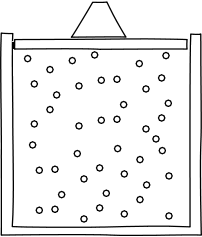All that seems reasonable enough. But you might also quite reasonably ask why the force of gravity just doesn't pull all the gas molecules down to the bottom of the cylinder, so that they lie in a big heap at the bottom of the cylinder, like a pile of dust. The simple answer looks at the gas microscopically and calls upon the thermal motions of the molecules to scatter them through the chamber. The relevant effect of these microscopic motions can be redescribed macroscopically as a pressure. The many microscopic collisions of the molecules with the piston, for example, appear macroscopically as a smooth pressure exerted by the gas on it.

Correspondingly the tendency of the gas to scatter upward because of the microscopic motions appears macroscopically as a pressure gradient in the gas. There is a higher pressure lower in the cylinder and that higher pressure tends to push the gas upward. Now different pressure gradients in the gas will lead to different density distributions, with equilibrium arising when the pressure gradient exactly balances the weight of the gas and piston above. Which pressure gradient will lead to a distribution proportional to exp(-mgh/kT) in every case? Well--you know the answer. It is exactly the pressure gradient given by the ideal gas law, PV=nkT!

To summarize, the assumption that a gas consists of many, independent, localized molecules leads to the ideal gas law. And it should come as no surprise that the argumentation can be reversed. If we have any gas in the context of Maxwell-Boltzmann statistical physics that satisfies the ideal gas law, then it consists of many, independent molecules.

There remains one subtle point that will become of central importance. The ideal gas law follows from the assumption that the gas consists of many, independent, localized molecules. Notice what is not assumed. It is not assumed that the molecules move in straight lines at uniform speed between collisions with other molecules; or that the molecules are the only matter present. The ideal gas law is a much more general result. It holds for any thermal system consisting of many, independent, localized components; and the notion of component and its context can be quite broad.

All this can be made precise mathematically with only a little more effort. See how here.

## 3. Einstein's Doctoral Dissertation

Of his statistical papers on 1905, the light quantum paper was published first. However in terms of the development of their ideas, Einstein's doctoral dissertation presents the natural starting point. The common ideas of the three papers appear in it in their simplest form and they are developed adventurously in the other two papers.

The point of Einstein's doctoral dissertation, "A New Determination of Molecular Dimensions," was clearly stated in its title. It was to determine how large molecules are. The answer was given in a particular way. A basic result of chemical atomism is that there are always the same number of molecules in one gram mole of any substances--such as 2g of hydrogen gas, or 18g of water, or 32g of oxygen gas. That number is N. It is called Avogadro's number in the English tradition and Loschmidt's number in Einstein's German tradition. Finding N then automatically tells us the mass of hydrogen molecules, water molecules and oxygen molecules.

The method Einstein hit upon was simple in conception. Pure water has a certain viscosity that measures how readily it flows. Water's viscosity is very much less than honey, for example, which flows much less readily. The addition of sugar to water to make a syrup like honey increases the viscosity. Einstein proposed that, at least in the case of dilute sugar solutions, the increase in viscosity is simply due to the bulk of the sugar molecules obstructing the free flow of the dissolving water. Einstein's project was to model this obstructive effect as a mathematical problem in fluid flow; and to compare the results with experimentally determined viscosities of dilute sugar solutions; and thereby to estimate N. The idea was simple, but its execution was not.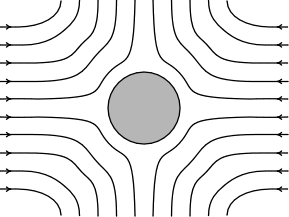Einstein managed to reduce the problem to computing the flow that results in the situation shown opposite. Water flows inward on one axis and then diverges outward on others. That flow will be impeded by the presence of a sugar molecule at the center, where the molecule is presumed to be a perfect sphere. That impeding of the flow, Einstein assumed, would manifest as an increase in the viscosity of the solution.

After a long and hard calculation, after Einstein had made many special assumptions just so that the computation could be done at all, Einstein arrived at his result. The apparent viscosity mu of the water was increased to mu* of the solution in direct relation to the fraction of the volume phi of the solution taken up by the sugar:

(1)     mu* = mu . (1 + phi)

And the fraction of the volume taken up by the sugar could be determined by simple geometry from rho the sugar density, m the molecular weight of the sugar, P the radius of the sugar molecule and N:

(2)     phi = (rho/m) . N . (4pi/3) . P3

Well, it was a little more complicated. Einstein made an error in the calculation and the correct result was
mu* = mu . (1 + (5/2)phi). The examiners did not notice. Einstein was awarded his PhD and years later corrected the mistake.

Don't be put off by all the terms in equations (1) and (2). All that really matters is that Einstein has equations that relate things that can be measured (viscosity of sugar solutions, etc.) to the thing he wants to know N. So Einstein could take equations (1) and (2), combine them and turn the outcome inside out. The result is

(3)     N = (3m/4 pi rho) . (mu*/mu) . 1/P3

Or, if we express it in terms that matter:

(3)     N = (things that can be measured) x 1/P3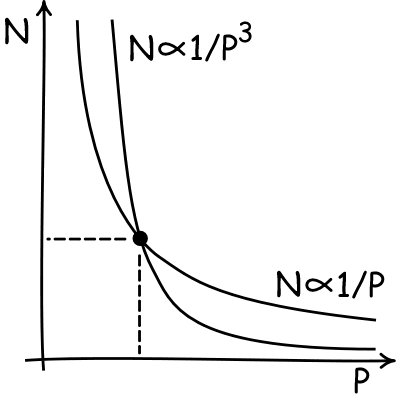You'll immediately see the problem with equation (3). N and the radius of the sugar molecule P are both things that we don't know (and want to know). So Einstein has that old foe of algebra homework: ONE equation in TWO unknowns. And we all learned in school that you cannot solve that. In effect we have a rule such that if we know the value of one unknown--P say-- we can figure out the other--in this case N. That is shown in the plot. We have a curve that displays all the values of P and the corresponding values of N that go with them. What Einstein needed was a second equation, so he would have TWO equations in TWO unknowns. Then he would have a second curve on the plot and where the two curves crossed he would find the unique values of both N and P.

But where could Einstein get his second equation? He found it by looking at how sugar diffuses in water. How he analyzed this diffusion process will be our real focus. So let me just state his result for the moment. It uses the diffusion coefficient D that determines how fast sugar diffuses and is measurable directly in experiment, and the ideal gas constant R.

(4)     N= (RT/6 pi mu D) . 1/P

or in terms of what matters

(4)     N = (things that can be measured) x 1/P

So Einstein now had two equations (3) and (4) in his two unknowns, N and P, and they could be solved. He found N = 2.1 x 1023. Later, after he corrected his calculation for his error, he had N = 6.6 x 1023, which is much closer to the modern value of 6.02 x 1023.

## 4. The Statistical Physics of Dilute Sugar Solutions

Diffusion is a familiar process. The smell of last nights pepperoni pizza soon fills the refrigerator as the aroma diffuses into every corner. Similarly a spoonful of sugar syrup carefully placed at the bottom of a cup of water (and not stirred!) will slowly diffuse over a period of days and weeks through the water making a (roughly) uniform sugar solution. The microscopic mechanism of diffusion is simply the scattering of sugar molecules under their random thermal motion. Indeed in dilute solutions, the sugar molecules form a system of a large number of molecules that do not interact with oneanother--they are widely spaced in the water because of the high dilution.A large number of molecules that do not interact?! This is exactly the condition that we saw the molecules of an ideal gas had to obey in order for the ideal gas law to obtain. So it should hold here as well. And it does! The random, microscopic motions of sugar molecules that leads to diffusion can be redescribed on a macroscopic level as a pressure, just as is the case with an ideal gas. This pressure is the familiar osmotic pressure so important in cell biology. Consider a semi-permeable membrane that can pass water but not sugar, such as the membrane in the figure opposite or a cell wall. The (gray) water can pass freely through it, but sugar molecules (the little white spheres) cannot. Through their collisions with the membrane, the sugar molecules exert a pressure on the membrane and the considerations that fix the size of the ideal gas pressure are exactly the same as those that fix the size of the osmotic pressure. The osmotic pressure P exerted by n sugar molecules in a volume V of water in dilute solution obeys the ideal gas    PV = nkT

 This osmotic pressure became central to Einstein's derivation of the result (4) for sugar diffusing in solution. To generate it, he imagined the same set up as I have described above, dissolved sugar molecules in a gravitational field. There are two processes acting on the sugar molecules. First, the effect of gravity is to pull the molecules downward. So they fall, as shown. A standard law in fluid mechanics, Stokes' law, expresses just how fast they fall under the pull of gravity.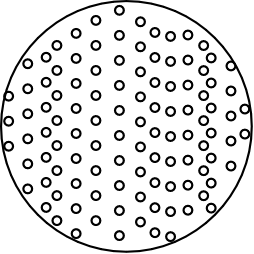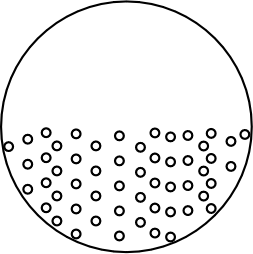Second, a diffusion process scatters the falling sugar molecules. Its net effect is to send the sugar molecules from regions of high concentration to regions of low concentrations. That precludes the falling molecules accumulating too much at the bottom of the vessel. Einstein used the fact that dissolved sugar exerts an osmotic pressure to determine the magnitude of this effect. The falling sugar forms a density gradient. The ideal gas law asserts that pressure is proportion to density, so there is an osmotic pressure gradient. And that pressure gradient drives the sugar back up. An equilibrium between the processes will be established when the amounts of sugar transported by the two processes in opposite directions are equal. The equation that sets those two rates of transport equal turns out to be just the second equation Einstein needed for the argument of his doctoral dissertation: (4)     N= (RT/6 pi mu D) . 1/P or in terms of what matters (4)     N = (things that can be measured) x 1/P

## 5. Einstein's Brownian Motion Paper

The argument and method of Einstein's dissertation was indirect and cumbersome. Since the original project of examining the viscosity of sugar solutions yielded one equation in two unknowns, he needed to introduce analysis of a second sort of physical process, diffusion, in order to get a result. To recall, he ended up with TWO equations in TWO unknowns, N and P, the radius of a sugar molecule:

(3)     N = (things that can be measured) x 1/P3

(4)     N = (other things that can be measured) x 1/P

We could well imagine Einstein examining these two unknowns, N and P, and lamenting that both are inaccessible to direct measurement. In the case of sugar solutions, of course, the problem is inescapable. To know one is to know the other; if we are ignorant of one we do not know the other. But wait--what if we were to apply this same analysis not to sugar solutions but to other solutions whose "molecules" are so big that we might measure their size directly under the microscope? That could be done. All we are really considering is a suspension in water of very finely divided particles, perhaps even like the tiny pollen grains Brown had observed under the microscope earlier in the 19th century. For these systems, there now only ONE unknown, N. Thermal motions would lead such particles to diffuse through water and, using equation (4) alone, Einstein could determine N from the measured rate of their diffusion.

I do not know if this is the reasoning that brought Einstein from the reflections of his doctoral dissertation to the Brownian motion paper. But I can say that the path is obvious and direct, just as it leads to a very much more adventurous result. Einstein is not longer computing the size of molecules, he has found a process which it seems that only a molecular kinetic theory of heat can accommodate!

 The remarkable fact is that Einstein could use exactly the same analysis for this process as he had used for the diffusion of sugar. The suspended particles consist of a large number of independent components--that you can see them under the microscope does not alter that fact. So they will exhibit thermal motions which in turn exert a pressure on a membrane that does not allow them to pass. At this point, no more calculation is needed. The particles will establish an equilibrium distribution in the gravitational field exactly as did the sugar molecules. Once again we can characterize that equilibrium by equating the rate at which the particles fall under gravity with the rate at which diffusion scatters them back up. The result is: (4)     N= (RT/6 pi mu D) . 1/P as before. Since P is now observed, all Einstein needs is to measure the rate of diffusion of the particles to recover D and then use (4) to compute N.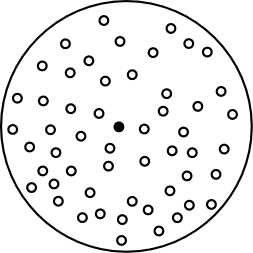This last step of the computation of N proved the most interesting. The thermal diffusion of these particles would manifest under the microscope as a random jiggling motion. Indeed Einstein conjectured that this was just the motion Brown has noted for pollen grains, although in this first paper Einstein lamented that he did not have enough data to be sure. For particles of size 0.001mm, Einstein predicted a displacement of approximately 6 microns in one minute.

## 6. The Importance of Einstein's Analysis of Brownian Motion

Following the easy logic of the pathway from his dissertation, we may overlook the momentous importance of what has just transpired. Einstein had found an effect that settled one of the major debates of the early 20th century!

In the course of the latter part of the 19th century, Maxwell, Boltzmann and others had struggled to establish that their statistical treatment of thermal processes deserved a place in physics. It was a difficult struggle. For their statistical accounts seemed to be at odds with established thermodynamics, grounded squarely in experiment. Most notoriously, there were (then) two laws fundamental laws of thermodynamics. The second law, the entropy principle, expressed the notion that thermodynamic processes were directed in time. Gases spontaneously expand to fill space. They do not spontaneously contract. In the statistical approach, however, they do spontaneously contract, but with very small probability. (We will see more of this shortly!) So Boltzmann struggled to establish that this basic law of thermodynamics only held with very high probability.

For Maxwell and Boltzmann, the project was to catch up with thermodynamics and show that they could do what the thermodynamicists were already doing without calling upon stories about atoms. Seen in this light, the opposition of energeticists like Ostwald at the start of the 20th century to atoms is quite understandable. They did not seem to need atoms to do their physics; and presuming atoms required compromising the basic laws of thermodynamics. So why play with the notion of atoms when it brought pain but no gain?

Einstein now had found a way to turn the tables. The strength of the thermodynamicists was their grounding in experiment. Yet here was an experimental effect--the random thermal motions of suspended particles--that could not be accounted for by ordinary thermodynamic means. One had to resort to something like a molecular kinetic account. Einstein pointed to this momentous outcome in rather dry language in the introduction to his paper:

"If it is really possible to observe the motion discussed here ... then classical thermodynamics can no longer be viewed as strictly valid even for microscopically distinguishable spaces, and an exact determination of the real size of a mole becomes possible."

Here I follow Anne Kox's analysis of Einstein's "eine exakte Bestimmung der wahren Atomgroesse" and translate Atomgroesse as size of a mole.

In addition to this foundational issue, there was a second theoretical bounty emerging from Einstein's analysis of Brownian motion. In order to determine N, Einstein needed to estimate the diffusion coefficient associated with the random motion of the suspended particles. This required a statistical analysis of the random jiggling of the particles.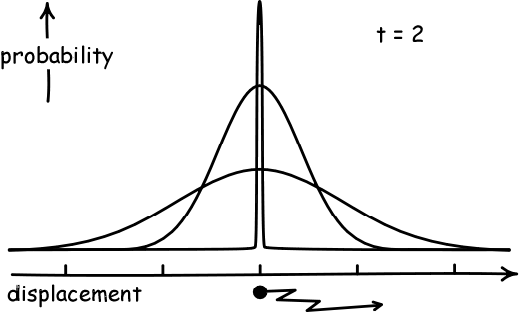The analysis had to be probabilistic. If a particle starts at some known position, we can at best specify the probabilities of it straying ever further from that initial point. The curve representing these probabilities is the familiar bell curve. As time t passes it becomes more and more flattened, capturing the greater probability of the particle straying from its initial position. Einstein showed that this flattening of the curve is directly related to the diffusion coefficient D. That is, the mean square displacement is 2.D.t. Through this analysis, Einstein's paper became one of the first treatments of the problem of the "random walk" and one of the founding documents in the new field of stochastic processes.

Finally there were some interesting subtleties in this random motion. First, the jiggles observed under the microscope were not the result of collisions with individual water molecules. You might presume that the effect of very many collisions with water molecules would rapidly average out to no effect at all. That turns out to be mistaken. The statistical analysis shows that even very many molecular collisions leaves a residual jiggle. Second, it is futile to try to find the average speed of the jiggling particles. Speed is displacement/time. Einstein's analysis shows that the average displacement is proportional to the square root of time. So the ratio of displacement/time varies as 1/(square root of time) and so goes to zero as time gets large. So if we try to average out the jiggles to find an average speed, we end up with averages that will get closer and closer to zero the longer the time period we consider.

## 7. The Light Quantum Paper: Einstein's Astonishing Idea

The great triumph of 19th century physics had been Maxwell's electrodynamics. It established definitively the wave character of light, identifying it as propagation in the electromagnetic field. It seemed impossible in the face of Maxwell's great achievement that we could ever go back to a view of light such as Newton held, that light consists of little corpuscles. Yet exactly this was the astonishing idea of Einstein's 1905 light quantum paper.

Einstein had several bases for this idea. Some were grounded directly in experiment. For example, he argued that we could best account for the photoelectric effect if we assumed that the energy of propagating light was spatially localized in little packets of size hf, where h is Planck's constant and f is the frequency of the light. This explanation of the photoelectric effect was cited in the awarding of the Nobel Prize to Einstein in 1921: "for his services to Theoretical Physics, and especially for his discovery of the law of the photoelectric effect."

The core argument of Einstein's paper was different, however. It drew on the thermodynamic behavior of high frequency heat radiation. What Einstein noticed was that there was an atomistic signature in its macroscopically measurable thermal properties. He noted that high frequency heat radiation behaved thermodynamically as if it consisted of independent, spatially localized quanta of energy of size hf. This remark was the light quantum hypothesis.

The idea that the macroscopic properties of a system may reveal its microscopic properties is not new. Indeed it has been present throughout the discussion so far. That the system exerts a pressure governed by the ideal gas law is just such a signature. It tells us that the system consists of many, independent components and this signature can be found in ideal gases, in dilute solutions and in systems of suspended particles. It actually turns out to be present in high frequency heat radiation as well. However its presence is harder to see. Heat radiation does exert a pressure, known as radiation pressure. That pressure is a function of the temperature and frequency of the radiation only. So we may well wonder how the ideal gas law PV=nkT could apply to it, for the ideal gas law clearly allows a volume dependence through the presence of the term V.

It turns out the the ideal gas law still does apply to a high frequency heat radiation. That fact is obscured by a novelty of heat radiation. The number of quanta in heat radiation is not fixed in the way the number of components is fixed for other systems such as an ideal gas. If we correct for that effect, compatibility with the ideal gas law is restored.When an ideal gas undergoes a constant temperature expansion, the ideal gas law PV=nkT tells us that the product of pressure and volume PV stay the same. That is, the pressure decreases and the volume increases. This is how we are used to seeing the ideal gas law manifested.

 When a system of high frequency heat radiation expands at constant temperature, new energy quanta are created in direct proportion to the volume V. That is, n/V remains constant. The idea gas law now tells us that the pressure P remains constant since we may write the law as P=(n/V)kT. The immediate effect is that the satisfaction of the ideal gas law is obscured since we are so used to the law telling us that pressure P decreases in a constant temperature expansion. The atomic signature is there; but it is in an unfamiliar form.## 8. A New Atomic Signature

Einstein did not mention the ideal gas law as an atomic signature for heat radiation. He did however demonstrate the existence of another atomic signature to which high frequency heat radiation did conform. He first illustrated that signature for the familiar case of an ideal gas.

The statistical approach to gases differed from a purely thermodynamic one, as noted above, in that it allows for gases to spontaneously recompress, albeit with very small probability. The analysis is very simple. Consider an ideal gas with just four molecules. The molecules will move randomly through the chamber shown and will mostly be spread throughout it.There is a probability of 1/2 that any given molecule will be in the left half of the chamber when we check. So the probability that all four of them will be there is just

(1/2) x (1/2) x (1/2) x (1/2) = (1/2)4.

The key fact of independence is what allows us just to multiply all four probabilities together to get the result. If we had n molecules, the probability would be

(1/2) x (1/2) x (1/2) x ...(n times)... x (1/2) = (1/2)n.

Since ordinary samples of gas will have of the order of n = 1024 molecules, this probability is fantastically small and we have no chance of observing this fluctuation in ordinary life. (And that is fortunate, for otherwise our lives in the air would like a small cork tossed about on a stormy sea!)

However the probability of this fluctuation is still quite definite. An ideal gas can spontaneously compress to half its volume with miniscule probability (1/2)n.

Statistical physics happens to give us another way to determine this probability, without us actually having to see the spontaneous recompression. The probability of the transition is related to a macroscopic thermodynamic quantity, entropy. We need not here go into many details of the nature of this quantity. All that matters for us is that entropy is a thermodynamic property of thermal systems, just as is energy, and its value is routinely given in tables of thermal properties of substances. I will not pause here to rant about the unfortunate mythology of mystery that surrounds the notion. A good part of it is due to plain old foggy thinking. See my website, http://www.pitt.edu/~jdnorton for details.

### The Simplest Version of the Argument

The details of the next steps of Einstein's argument are a little messy for people who don't like logarithms. So here's the very simplest version without logarithms.

The thermodynamic quantity entropy tells us what sorts of transformations thermal systems will undergo. The basic rule is that thermal systems will tend to states of higher entropy. So the entropy difference between two states of a system gives us information on the tendency of the system to move between the states. Indeed the "tendency" can be given a quite precise measure as a probability. If we know the entropy difference between two states of a system, we know the probability that the system will spontaneously move between those two states.

 Now recall that the entropy of a system is an ordinary thermodynamic quantity like energy. Just as you can measure the energy content of some volume of radiation by a suitable experiment, you can also measure the entropy content of that system. To get a sense of how it works, imagine that you slowly heat some system which is initially at some absolute temperature T. You can figure out how much the energy of the system changes with each unit of heat you add--one unit of energy is added for each unit of heat. The corresponding calculation for entropy is almost as simple. For each unit of heat energy you add when the system is at T, you add 1/T units of entropy.

That is just what Einstein did for heat radiation. More precisely, he took other people's measures of entropy and used them to figure out the entropy difference between two states: a quantity of heat radiation of energy E at one, particular high frequency f and a second quantity of heat radiation of the same energy E and frequency f, but half the volume.

From the entropy change between those two states, Einstein could infer that the probability of the quantity of radiation spontaneously fluctuating to half its volume is just (1/2)(E/hf). Written out more fully that is

(1/2) x (1/2) x (1/2) x ...(E/hf times)... x (1/2) = (1/2)(E/hf)

Comparing this formula to the corresponding formula for n molecules, it is almost impossible to avoid concluding that this quantity of high frequency radiation consists of E/hf spatially localized radiation molecules--Einstein called them "light quanta"--that move independently through the volume.

The picture to have in mind is:The best part is that the probability formula tells us directly how big these light quanta are. The probability comes from multiplying E/hf factors of (1/2) together. So we infer that the total energy E of the radiation is divided into that many quanta of energy, each of size hf.

### The Fancier Version of the Argument

Now here's the fancier version.

The entropy change between two states S is related to the logarithm of the probability W of a spontaneous transition between the two states by the formula

S = k log W
.

Einstein judged this result so important that he named it "Boltzmann's Principle." That wonderful formula was engraved on Boltzmann's gravestone; it is the bridge we need between the macroscopic and the microscopic.

Apply this principle to the case of the ideal gas of n molecules that spontaneously compresses to half its volume with probability W = (1/2)n. We find that the difference in entropy between the gas and that same gas occupying one half the volume is given by

(5)     S = k.log W = k.log (1/2)n = - nk.log 2

While we arrived at this entropy difference by thinking about extremely improbable fluctuations in the gas' volume, it can also be found in standard thermodynamic treatises, derived entirely from macroscopic properties of ideal gases, without any mention of microscopic properties and very unlikely events. (In particular, you do not need to know the size of N to get this formula. For nk = nm.R, where nm is the number of moles and R is the ideal gas constant.) But now that we know how to read the logarithmic dependence of entropy on volume of (5), we can recognize it as a macroscopic signature of the spatially localized, independent atoms in the ideal gas.

Einstein recognized this same signature in a single frequency cut of high frequency heat radiation. By drawing directly on experimental measurements of the thermal properties of high frequency heat radiation, he noted that the entropy difference between two quantities of radiation of energy E and frequency f, one at the full volume and one at the half volume, is just:

(6)     S = - (E/hf).k.log 2 = k log (1/2)(E/hf)

The analogy between formulae (5) and (6) is obvious.

Einstein had now found the macroscopic signature of atoms in high frequency heat radiation. Comparing equations (5) and (6), we immediately see that the heat radiation is governed by a formula appropriate to a system consisting of E/hf independent components. Or, to put it another way, it is as if the energy E of the radiation is divided into independent, spatially localized components of energy hf. This, you will recall, is just Einstein's light quantum hypothesis, but now read from equations (5) and (6).

You should note how carefully hedged Einstein's statement of the light quantum hypothesis is. Its most careful formulation from his 1905 paper is:

"Monochromatic radiation of low density behaves--as long as Wien's radiation formula is valid [i.e. at high values of frequency/temperature]--in a thermodynamic sense, as if it consisted of mutually independent energy quanta of magnitude [hf]."

Einstein is very careful to add many conditions: high frequency/temperature, low density, "as if" and "in a thermodynamic sense."

That caution is very prudent. Einstein had not explained away the quite prodigious body of evidence from the 19th century all pointing to the wavelike character of light. Indeed that evidence will never go away. What Einstein eventually decided a few years later is that both wave and particle characters are needed for a full account of light. Sometimes light will behave like a wave; sometimes like a localized particle; and sometimes both. That we now know as "wave-particle" duality.

Modern readers often find it irresistible to jump from these light quanta of 1905 to modern photons; that is, to imagine that Einstein was just proposing that light really consists of particles or corpuscles after all. That would be a risky jump for all the reason just given. In addition, an essential part of the notion of a photon is that it carries momentum. Nothing in Einstein's arguments so far have established that his light quanta of 1905 also carry momentum. That conclusion had to be established by further analysis and it came with time.

## 9. Conclusion

Einstein published three papers in statistical physics in 1905. By any measure, their content is extraordinary. In one form or another they contained the seeds of the new theorizing in statistical physics of the twentieth century. They provided a new method of estimating the size of molecules, a treatment of the diffusion of solutes and small particles in viscous media, the identification of a phenomenon that turned the tide of resistance to molecular kinetic methods in physics, a foundational analysis in the new field of stochastic processes and the demonstration of the granular character of electromagnetic radiation.

When faced with this wealth, it is hard not to be awed, let alone to find a unifying theme that permeates the work. My goal has been to display just such a theme, even if the theme does not pass through the heart of every aspect of Einstein's achievement. That theme is the simple idea that thermal systems consisting of many, spatially localized, independent components have the same macroscopic properties, most notably the satisfaction of the ideal gas law. This fact simplifies analysis of many systems, since once the independence of the components is known, the ideal gas law must follow, whether the system is a gas, dilute solution or microscopically visible particles in suspension. And the inference can be inverted. Once an atomic signature is seen, one can infer back to the constitution of the system. In the case of high frequency heat radiation, the presence of the atomic signature was so definite that it emboldened Einstein to overthrow the great achievement of 19th century physics. He rejected Maxwell's electrodynamics and its wave theory of light, in favor of a new and still ill-formed quantum account of radiation.

Copyright John D. Norton, May 8, 2005. Minor corrections, May 15, 2005; link to "How big is an atom? June 17, 2006. Section 8 revised April 11, 2007.